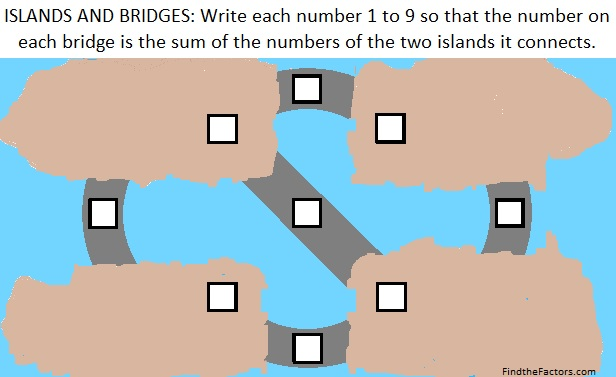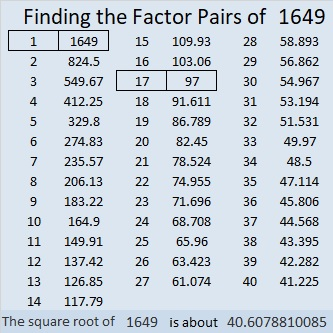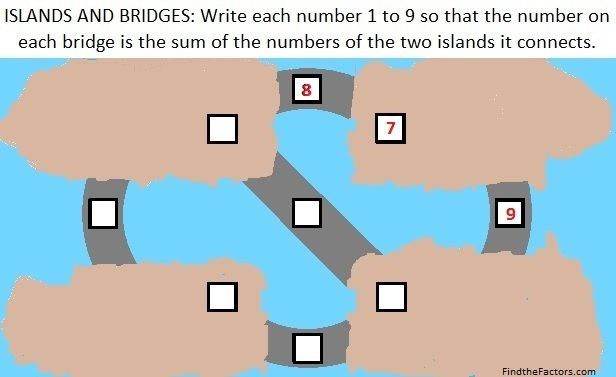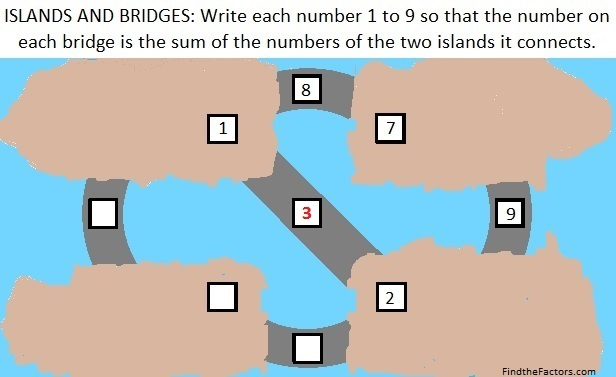# 1649 Tweaking a Puzzle Posed by Sunil Singh @Mathgarden

Contents

### Today’s Puzzle:

A couple of days ago on Twitter, I saw an interesting puzzle posed by Sunil Singh @Mathgarden.

After I found one of several of its solutions, I wondered if I could add a bridge that would use all nine numbers from 1 to 9 in the solution, so I tweaked it. I decided to move the puzzle to the ocean when I added that extra bridge.I was able to solve this problem using logic and addition facts, rather than algebra. Try solving it yourself. If you want to see any of the steps I used to solve the puzzle, scroll down to the end of the post.

### Factors of 1649:

• 1649 is a composite number.
• Prime factorization: 1649 = 17 × 97.
• 1649 has no exponents greater than 1 in its prime factorization, so √1649 cannot be simplified.
• The exponents in the prime factorization are 1 and 1. Adding one to each exponent and multiplying we get (1 + 1)(1 + 1) = 2 × 2 = 4. Therefore 1649 has exactly 4 factors.
• The factors of 1649 are outlined with their factor pair partners in the graphic below.### More About the Number 1649:

1649 is the sum of two squares in TWO different ways:
32² + 25² = 1649, and
40² + 7² = 1649.

1649 is the hypotenuse of FOUR Pythagorean triples:
399-1600-1649, calculated from 32² – 25², 2(32)(25), 32² + 25²,
560-1551-1649, calculated from 2(40)(7), 40² – 7², 40² + 7²,
776 1455 1649, which is (8-15-17) times 97, and
1105 1224 1649, which is 17 times (65-72-97).

### Some Logical Steps to Solve Today’s Puzzle:

I found four different ways to write the solution but they are all rotations or reflections of each other. Here are the steps to one of those four ways:

1st Step: The biggest number that can be used is 9. Since every island must be included in at least two sums, neither 8 nor 9 can be an addend; they both must be sums. 7 must be an addend in their sums because adding any number to 7 will yield 8, 9, or some larger forbidden number. Thus 8 and 9 are bridges that connect to island 7.I chose to write 7, 8, and 9 in the top right section, but 8 and 9 could change places with each other. I could have also chosen to write those numbers in the bottom left area.

2nd Step: 1 + 7 = 8, and 7 + 2 = 9.3rd Step: 1 + 2 = 3.4th Step: The last island must be the smallest remaining number (4) because the smallest remaining number can’t be the sum of a bigger number and the number on either adjacent island.Final Step: 1 + 4 = 5, and 4 + 2 = 6.Did you enjoy this puzzle? How did my steps compare to the steps that you took?

1.Denise Gaskins
2.ivasallay